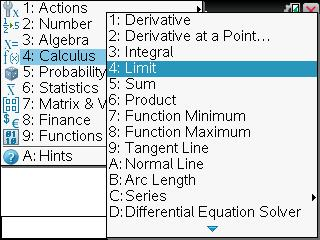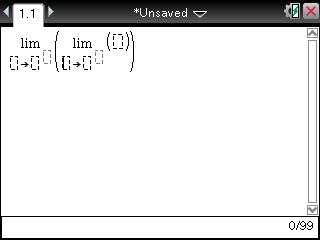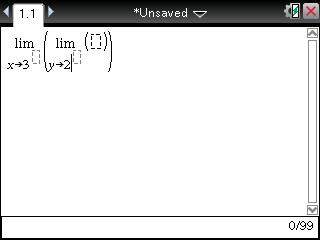# Knowledge Base

## Solution 30371: Calculating Double Limits on the TI-Nspire™ CAS Family Products.

### How do I calculate double limits on the TI-Nspire CAS family products?

To calculate double limits using the TI-Nspire CAS family products, the "Calculator" or "Scratchpad" application and "Limit" function must be used. Follow the steps below to calculate the double limit for the example function.

Example: Find the double limit of the function (x+1)/(y+5) as x approaches 3 and y approaches 2.

1) Press [home] and select the Calculator or Calculate (from the Scratchpad) application.
2) Press [menu]   to select the Limit function. The Limit template is displayed.3) The handheld will prompt for the variable in the limit. From the example function, input x and press the [right arrow] key.
4) The handheld will prompt for the value that the limit will approach. Input 3 and press the [right arrow] key.5) Repeat the 3rd and 4th steps above using y as the variable in the limit and 2 for the value the limit will approach.6) The handheld will prompt for the direction of the limit (left, right, or both). For this example, leave it blank and press the [right arrow] key.
7) Input the example function (x+1)/(y+5).
8) Press [ENTER]. The handheld should return .571429 as the solution.

For more information on how to use the TI-Nspire CAS handheld, please see the TI-Nspire CAS guidebook.